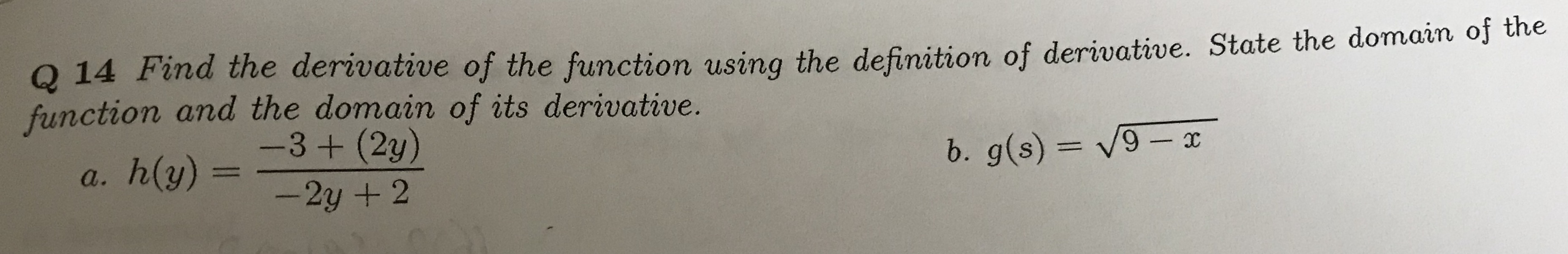# Q 14 Find the derivative of the function using the definition of derivative. State the domain of the function and the domain of its derivative. -3 + (2y) b. g(s)= V9- x h(y) = a. -2y + 2

Questionhelp_outlineImage TranscriptioncloseQ 14 Find the derivative of the function using the definition of derivative. State the domain of the function and the domain of its derivative. -3 + (2y) b. g(s)= V9- x h(y) = a. -2y + 2 fullscreen